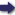(London :  Kegan Paul, Trench, Trübner & Co.,  1910.)

 Tools

## Search this bookPrev Page 37 Next``` CHAPTER LII. 37 would not be necessary to double the remainder. But the inventor of this method seems to have jDreferred the reduplication in order to get smaller numbers; for if we reckon with single adhimdsas, we get the fraction of -g-fff^, which may be reduced by 96 as a common divisor. Thereby we get 89 as the multiplicator, and 5400 as the divisor. In this the inventor of the method has shown his sagacity, for the reason for his computation is the intention of getting partial lunar days and smaller multiplicators. His method (i.e. Brahmagupta's) for the computation Method for of the Unardtra days is the following :— ta\lon™f^t^i'o If we divide the universal lunar days by the uni- daysaocord- versal ilnardtra days, we get as quotient 63 and a ^rihma- fraction, which mav be reduced bv the common divisor s^p"^^- •^ "^ . Page 221. 450,000. Thus we get 634|;ff f lunar days as the period of time within which one unardtra day sums up. If we change this fraction into eleventh parts, we get y\ and a remainder of f|-;-f ^4, which, if expressed in minutes, is equal to o' 59" 54"'. Since this fraction is very near to one whole, people have neglected it, and use, in a rough way, -|[-J instead. Therefore, according to the Hindus, one unardtra day sums up in 63^^ or '^^^ lunar days. If we now multiply the number of unardtra days, which corresponds to the number of lunar days by 63lf,'fff) tihe product is less than that which we get by multiplying by 63^°. If we, therefore, want to divide the lunar days by ''^-{', on the supposition that the quotient is equal to the first number, a certain portion must be added to the lunar days, and this portion he (the author of Pulisa-Siddhdnta) had not computed accu¬ rately, but only approximatively. For if we multiply the universal unardtra days by 703, we get the product 17,633,032,650,000, which is more than eleven times the universal lunar days. And if we multiply the universal lunar days by 11, we get the product 17,632,989,000,000. ```Prev Page 37 Next